Anzeige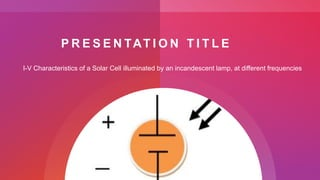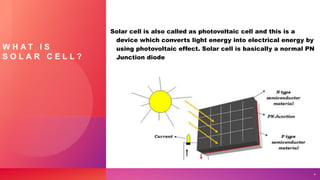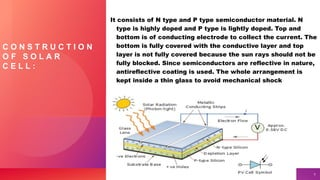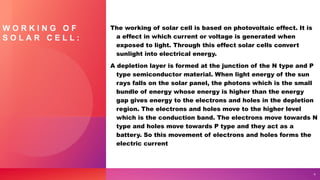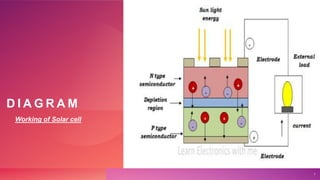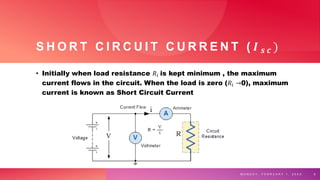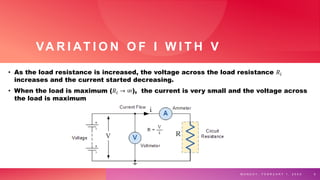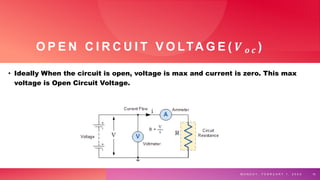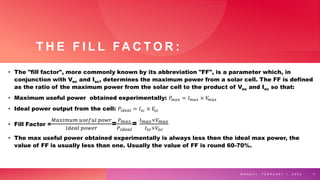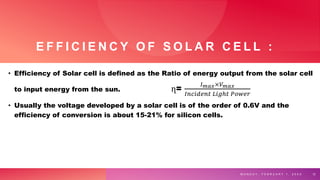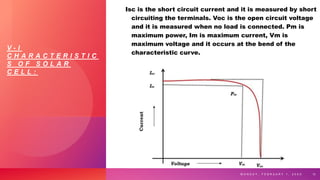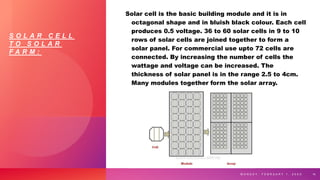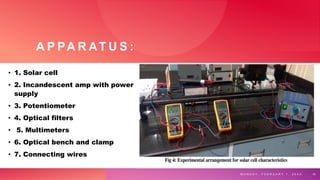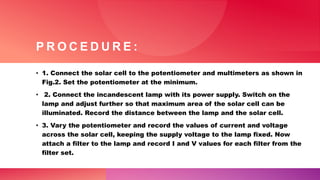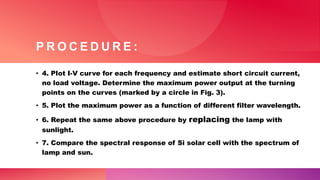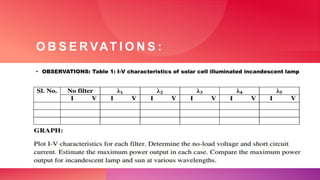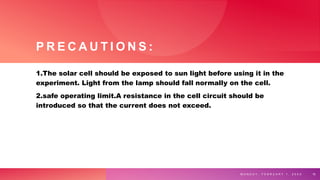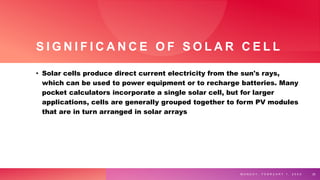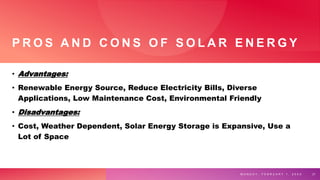1 von 22
Anzeige

1. M O N D A Y , F E B R U A R Y 1 , 2 0 X X 1
2. G R O U P N O # 0 2 1) M.Hassnain Haider (leader) 2) Ahamd Elahii 3) Junaid Anwar 4) Shazaib Asghar 5) Rafaqat Khokhar 6) Umer Khalil
3. P R E S E N TAT I O N T I T L E I-V Characteristics of a Solar Cell illuminated by an incandescent lamp, at different frequencies
4. W H A T I S S O L A R C E L L ? Solar cell is also called as photovoltaic cell and this is a device which converts light energy into electrical energy by using photovoltaic effect. Solar cell is basically a normal PN Junction diode 4
5. C O N S T R U C T I O N O F S O L A R C E L L : It consists of N type and P type semiconductor material. N type is highly doped and P type is lightly doped. Top and bottom is of conducting electrode to collect the current. The bottom is fully covered with the conductive layer and top layer is not fully covered because the sun rays should not be fully blocked. Since semiconductors are reflective in nature, antireflective coating is used. The whole arrangement is kept inside a thin glass to avoid mechanical shock 5
6. W O R K I N G O F S O L A R C E L L : The working of solar cell is based on photovoltaic effect. It is a effect in which current or voltage is generated when exposed to light. Through this effect solar cells convert sunlight into electrical energy. A depletion layer is formed at the junction of the N type and P type semiconductor material. When light energy of the sun rays falls on the solar panel, the photons which is the small bundle of energy whose energy is higher than the energy gap gives energy to the electrons and holes in the depletion region. The electrons and holes move to the higher level which is the conduction band. The electrons move towards N type and holes move towards P type and they act as a battery. So this movement of electrons and holes forms the electric current 6
7. D I A G R A M Working of Solar cell 7
8. S H O R T C I R C U I T C U R R E N T ( 𝑰 𝒔 𝒄 ) • Initially when load resistance 𝑅𝑖 is kept minimum , the maximum current flows in the circuit. When the load is zero (𝑅𝑖 →0), maximum current is known as Short Circuit Current M O N D A Y , F E B R U A R Y 1 , 2 0 X X 8
9. VA R I AT I O N O F I W I T H V • As the load resistance is increased, the voltage across the load resistance 𝑅𝑖 increases and the current started decreasing. • When the load is maximum (𝑅𝑖 → ∞), the current is very small and the voltage across the load is maximum M O N D A Y , F E B R U A R Y 1 , 2 0 X X 9
10. O P E N C I R C U I T V O LTA G E ( 𝑽 𝒐 𝒄 ) • Ideally When the circuit is open, voltage is max and current is zero. This max voltage is Open Circuit Voltage. M O N D A Y , F E B R U A R Y 1 , 2 0 X X 10
11. T H E F I L L FA C T O R : • The "fill factor", more commonly known by its abbreviation "FF", is a parameter which, in conjunction with Voc and Isc, determines the maximum power from a solar cell. The FF is defined as the ratio of the maximum power from the solar cell to the product of Voc and Isc so that: • Maximum useful power obtained experimentally: 𝑃𝑚𝑎𝑥 = 𝐼𝑚𝑎𝑥 × 𝑉 𝑚𝑎𝑥 • Ideal power output from the cell: 𝑃𝑖𝑑𝑒𝑎𝑙 = 𝐼𝑠𝑐 × 𝑉 𝑜𝑐 • Fill Factor = 𝑀𝑎𝑥𝑖𝑚𝑢𝑚 𝑢𝑠𝑒𝑓𝑢𝑙 𝑝𝑜𝑤𝑟 𝐼𝑑𝑒𝑎𝑙 𝑝𝑜𝑤𝑒𝑟 = 𝑃𝑚𝑎𝑥 𝑃𝑖𝑑𝑒𝑎𝑙 = 𝐼𝑚𝑎𝑥×𝑉𝑚𝑎𝑥 𝐼𝑠𝑐×𝑉𝑜𝑐 • The max useful power obtained experimentally is always less then the ideal max power, the value of FF is usually less than one. Usually the value of FF is round 60-70%. M O N D A Y , F E B R U A R Y 1 , 2 0 X X 11
12. E F F I C I E N C Y O F S O L A R C E L L : • Efficiency of Solar cell is defined as the Ratio of energy output from the solar cell to input energy from the sun. ղ= 𝐼𝑚𝑎𝑥×𝑉𝑚𝑎𝑥 𝐼𝑛𝑐𝑖𝑑𝑒𝑛𝑡 𝐿𝑖𝑔ℎ𝑡 𝑃𝑜𝑤𝑒𝑟 • Usually the voltage developed by a solar cell is of the order of 0.6V and the efficiency of conversion is about 15-21% for silicon cells. M O N D A Y , F E B R U A R Y 1 , 2 0 X X 12
13. V - I C H A R A C T E R I S T I C S O F S O L A R C E L L : Isc is the short circuit current and it is measured by short circuiting the terminals. Voc is the open circuit voltage and it is measured when no load is connected. Pm is maximum power, Im is maximum current, Vm is maximum voltage and it occurs at the bend of the characteristic curve. M O N D A Y , F E B R U A R Y 1 , 2 0 X X 13
14. S O L A R C E L L T O S O L A R F A R M : Solar cell is the basic building module and it is in octagonal shape and in bluish black colour. Each cell produces 0.5 voltage. 36 to 60 solar cells in 9 to 10 rows of solar cells are joined together to form a solar panel. For commercial use upto 72 cells are connected. By increasing the number of cells the wattage and voltage can be increased. The thickness of solar panel is in the range 2.5 to 4cm. Many modules together form the solar array. M O N D A Y , F E B R U A R Y 1 , 2 0 X X 14
15. A P PA R AT U S : • 1. Solar cell • 2. Incandescent amp with power supply • 3. Potentiometer • 4. Optical filters • 5. Multimeters • 6. Optical bench and clamp • 7. Connecting wires M O N D A Y , F E B R U A R Y 1 , 2 0 X X 15
16. P R O C E D U R E : • 1. Connect the solar cell to the potentiometer and multimeters as shown in Fig.2. Set the potentiometer at the minimum. • 2. Connect the incandescent lamp with its power supply. Switch on the lamp and adjust further so that maximum area of the solar cell can be illuminated. Record the distance between the lamp and the solar cell. • 3. Vary the potentiometer and record the values of current and voltage across the solar cell, keeping the supply voltage to the lamp fixed. Now attach a filter to the lamp and record I and V values for each filter from the filter set.
17. P R O C E D U R E : • 4. Plot I-V curve for each frequency and estimate short circuit current, no load voltage. Determine the maximum power output at the turning points on the curves (marked by a circle in Fig. 3). • 5. Plot the maximum power as a function of different filter wavelength. • 6. Repeat the same above procedure by replacing the lamp with sunlight. • 7. Compare the spectral response of Si solar cell with the spectrum of lamp and sun.
18. O B S E R VAT I O N S : • OBSERVATIONS: Table 1: I-V characteristics of solar cell illuminated incandescent lamp
19. P R E C A U T I O N S : 1.The solar cell should be exposed to sun light before using it in the experiment. Light from the lamp should fall normally on the cell. 2.safe operating limit.A resistance in the cell circuit should be introduced so that the current does not exceed. M O N D A Y , F E B R U A R Y 1 , 2 0 X X 19
20. S I G N I F I C A N C E O F S O L A R C E L L • Solar cells produce direct current electricity from the sun's rays, which can be used to power equipment or to recharge batteries. Many pocket calculators incorporate a single solar cell, but for larger applications, cells are generally grouped together to form PV modules that are in turn arranged in solar arrays M O N D A Y , F E B R U A R Y 1 , 2 0 X X 20
21. P R O S A N D C O N S O F S O L A R E N E R G Y • Advantages: • Renewable Energy Source, Reduce Electricity Bills, Diverse Applications, Low Maintenance Cost, Environmental Friendly • Disadvantages: • Cost, Weather Dependent, Solar Energy Storage is Expansive, Use a Lot of Space M O N D A Y , F E B R U A R Y 1 , 2 0 X X 21
22. Sample Footer Text 22
Anzeige